Courses

# Testing Of Single Phase Transformers Electrical Engineering (EE) Notes | EduRev

## Electrical Engineering (EE) : Testing Of Single Phase Transformers Electrical Engineering (EE) Notes | EduRev

The document Testing Of Single Phase Transformers Electrical Engineering (EE) Notes | EduRev is a part of the Electrical Engineering (EE) Course Electrical Machines.
All you need of Electrical Engineering (EE) at this link: Electrical Engineering (EE)

Testing of Single Phase Transformers

In the last lecture, we have seen how to represent a single phase transformer by approximate equivalent circuit either refer to the primary or refer to the secondary. Now it would have been convenient if we can derive a single equivalent circuit without being too concerned about whether it is referred to the primary or referred to the secondary. There are some practical advantages of having equivalent circuit like that. The way we can do it is by converting this equivalent circuit to per unit equivalent circuit where each parameter of the equivalent circuit and each variable are expressed in power units. How do we do that?
In order to get per unit as equivalent circuit we have to define certain base quantities; although the bases can be selected arbitrarily the customer practice is to select the rated voltage of a given side of a transformer to be the base voltage. Therefore, the rated voltage of the primary will be the base voltage for the primary side variables and parameters.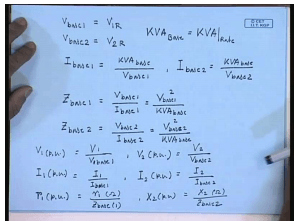So, we call it v base 1 equal to rated voltage of the primary. Similarly v base 2 would be the rated voltage of the secondary. Since in a transformer the KVA of the primary and the secondary are the same; therefore, there is only a single KVA base is the rated KVA of the transformer. From these bases we can define the base currents; I base 1 equal to KVA base divided by v base 1. Similarly, I base 2 equal to KVA base by v base 2. Next step we can define a base impedance z base 1 equal to v base 1 by I base 1, and this is the v base 1 square by KVA base, and z base 2 equal to v base 2 divided by I base 2 equal to v base 2 square by KVA base.
Once we define these base quantities voltage in per unit can be written as V 1 in per unit equal to the actual value of V 1 divided by V 1 base or rather v base 1. Similarly V 2 in per unit will be equal to actual value of V 2 divided by v base 2. I 1 in per unit will be equal to actual value of I 1 divided by I base 1. Similarly I 2 in per unit would be equal to I 2 divided by I base 2. Any impedance let us say r 1 in per unit would be equal to actual value of r 1 in ohms divided by z base 1, and let us say x 2 in per unit will be actual value of x 2 in ohms divided by z base 2. Now how does defining this base quantities and per unit values head to achieve a single unique equivalent circuit of a transformer let us see.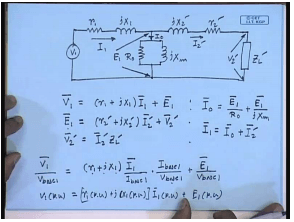For that let us consider the exact equivalent circuit of the transformer refers to the primary side. The set of equation that describes this equivalent circuit V 1 equal to r 1 plus j x 1 into I 1 plus E 1. E 1 equal to r 2 dash plus j x 2 dash I 2 dash plus V 2 dash, and V 2 dash equal to I 2 dash Z L dash. Similarly I 0 equal to E 1, these are all phasors, by R 0 plus E 1 by j x m. I 1 equal to I 0 plus I 2 dash. Now let us convert them to equations in per unit variables and parameters. In order to do that let us first divide the left hand side by the first equation by v base 1. So, it becomes v 1 by v base 1 equal to r 1 plus j x 1. This also divide I 1 by I base 1, and since also multiply by I base 1 divided by v base 1 plus E 1 by v base 1, but this is V 1 per unit equal to v base 1 by I base 1 is z base 1 and r 1 plus j x 1 by z base 1 is r 1 per unit plus j x 1 per unit into I 1 per unit plus E 1 per unit.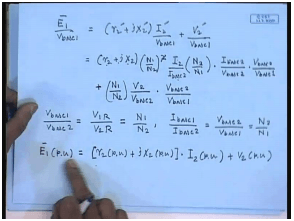Similarly, by dividing the second equation by v base 1 we get, the right hand side can be rewritten as but from our definition of the base quantities equal to N 1 by N 2, and also applying this relations we have E 1 per unit equal to r 2 plus j x 2 divided by z base 2; that is r 2 per unit plus j x 2 per unit multiplied by I 2 by I 2 base is I 2 per unit. N 2 by N 1 cancels with 1 of N 1 by N 2 square. So, this becomes N 1 by N 2 into v base 2 by v base 1 which is 1. N 1 by N 2 into v base 2 by v base 1 which is equal to 1. Therefore this is what we get from this, and on this side we have N 1 by N 2 into v base 2 by v base 1 which is again 1, and we are left with only V 2 per unit. So, we see that we arrive at the same equation in per unit.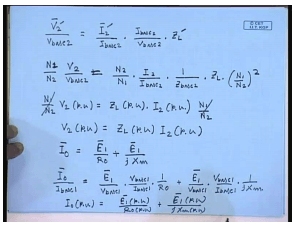Similarly, we can apply similar thing to the last equation V 2 dash bar by v base 2 equal to I 2 dashed bar by I base 2 into I base 2 by v base 2 into Z L dash, but V 2 dashed equal to N 1 by N 2 into V 2 divided by v base 2 equal to I 2 dash is N 2 by N 1 into I 2 divided by I base 2 into 1 by z base 2 into Z L into N 1 by N 2 square, or N 1 by N 2 V 2 per unit equal to Z L per unit into I 2 per unit into N 1 by N 2.
Cancelling N 1 by N 2 from both sides we have the per unit relation V 2 per unit equal to Z L per unit into I 2 per unit. Coming to the fourth equation I 0 equal to E 1 by R 0 plus E 1 by j x m, one can write I 0 by I base 1 equal to E 1 bar by v base 1 into v base 1 by I base 1 into 1 by R 0 plus E 1 bar by v base 1 into v base 1 by I base 1 into 1 by j x m or I 0 per unit equal to E 1 per unit divided by R 0 per unit plus E 1 per unit divided by j x m per unit.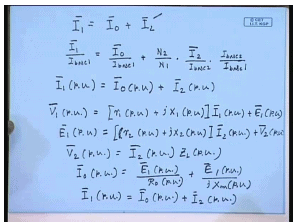For the last equation I 1 equal to I 0 plus I 2 dash. One can write I 1 bar by I base 1 equal to I 0 bar by I base 1 plus N 2 by N 1 I 2 divided by I base 2 into I base 2 by I base 1, or I 1 per unit equal to I 0 per unit plus I 2 per unit, since N 2 by N 1 into I base 2 by I base 1 is unity. Therefore, the complete set of equations in per unit can be written as E 1 per unit equal to r 2 per unit plus j x 2 per unit into I 2 per unit plus V 2 per unit. V 2 per unit equal to I 2 per unit into Z L per unit. I 0 per unit equal to E 1 per unit by R 0 per unit plus E 1 per unit by j x m per unit. And I 1 per unit equal to I 0 per unit plus I 2 per unit. This is a unit set of equation; the r 1 and r 2 expressed in per units with respect to their base are same on both sides.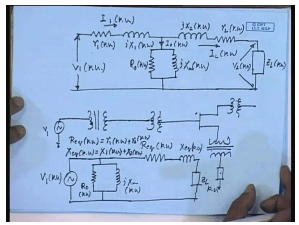Therefore the equivalents per unit equivalent circuit exact equivalent circuit will be similar to our familiar exact equivalent circuit except that now all parameters are to be expressed in per units, and all variables are also expressed in per units. And once they are expressed in per units there is no need to refer these parameters to one side or the other by any turns ratio. Now this is advantageous in many situations. It may not be so apparent when we consider a single transformer; however, it will be easy to understand if we have several such transformer. For example, in a power system, suppose we have a force V 1 which supplies some transformer supplies another transformer which may in turn supply few more transformers which may in turn supply the load.

In such a situation if we have to refer every impedance to a single side in order to solve the problem, it will be too complicated, and for very large power system with literally tens of thousands of transformer it will become completely impossible. Under such situation representing the parameters of the transformer or the load or the transmission line in per unit system is advantageous, because it does not involve referring the impedances to a single side. Therefore, for almost all practical calculation the transformer parameters are normally represented in per unit. In fact, just as with exact parameters the approximate equivalent circuit of the transformer can also be drawn in per unit where R e q per unit is simply r 1 per unit plus r 2 per unit; similarly, X e q per unit equal to x 1 per unit plus x 2 per unit.

Now a transformer equivalent circuit whether expressed in actual parameters referred to the primary or secondary are expressed in per unit parameter will be useful only if one knows the values of the parameter. Now therefore, it is important to be able to determine the transformer equivalent circuit parameters either from the design data or by carrying out certain tests. It is possible to estimate the parameters of the transformer from the dimensions and the design data; however, in most cases the user will not have access to such design data. And also the design data gives a first approximation of these parameters; in order to get more accurate values the user must conduct certain tests on the transformer.

There are two tests that are normally used to find out the equivalent circuit parameters of the transformer. One is no load test which finds outs the shunt branch parameters r 0 and x m are connected across the source. So, these are called the shunt branch parameters. Similarly, a short circuit test to find out the approximate equivalent circuit parameter R e q and X e q which are called the series branch parameter; we will see how these tests are done.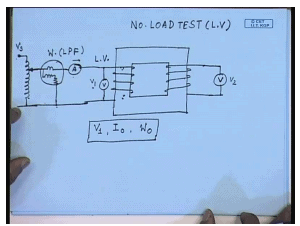Let us first concentrate on no load test. In order to perform a no load test you take the transformer under test; keep one side open connected to a voltmeter a. c. voltmeter. On the other side you connect an ammeter, a wattmeter, and normally supply it from what we call a single phase variac which can supply at least the rated voltage of this side of the transformer. In order to do the no load test the variac is started from zero voltage position, then the power is applied; the voltage supplied by the variac is slowly raised till the voltmeter connected on the other side reads the rated voltage of this side.

Under that condition you take the readings of voltage v; there is also another voltmeter connected here. Let us call it V1; this is V 2. You take the readings of V 1. This current I 0 which is the no load current and the wattmeter reading W 0. From this we will see how to find out the shunt branch parameters very shortly, but before that let us be a little more specific about how to choose this equipment and which side should we choose? Should we keep the high voltage side open and apply voltage to the low voltage side or the other way round? Now theoretically it does not make a difference. It can be used at, the supply can be given to either side of the transformer, and per unit parameters determined will be the same.

However there are some practical limitations. For example, this test is to be performed by applying rated voltage of the side; that is the V 1 should read the rated voltage of the transformer on this side. So, if this is the H V side then one, I will need a variac which can apply the rated high voltage across this transformer, and for high voltages this may be really large in kilovolts; that is one problem. The meters should also be rated at the high voltage winding rating of the transformer. The second problem is since the transformer is in open circuit condition it draws only no load current. Now the no load current of a well designed transformer is less than 5 percent of the rated full load current. As such the rated full load current of the high voltage side is much lower than that of the low voltage side.

No load current being even only 5 percent of the high voltage rated current its value will be really small, and it will be difficult to get an accurate n of ammeter to read that, since as we will see the same ammeter will also be required to read the rated current milliliter test. So, it is usually preferred to supply the voltage to the low voltage side of the transformer and keep the high voltage side open. Therefore, no load tests are usually performed on the low voltage side. One should be also careful about choosing the wattmeter.
The wattmeter potential coil should be rated at the rated voltage of the low voltage side of the transformer; however, the current flowing through the wattmeter current coil will only be the no load coil, no load current. Therefore, the current rating of the current coil of the wattmeter should be low; not only that at no load the transformer per factor is very poor, it is almost 0. So, one must use a low per factor wattmeter LPF wattmeter to get reliable readings. Now let us see how we use the no load test data to find out the shunt branch parameters at no load.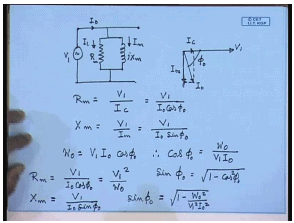The transformer equivalent circuit the approximate equivalent circuit of the transformer looks like this. Since the load is open, these terminals are open. We are applying voltage here voltage of V 1 here; the current drawn is I 0, and the power reading is w 0. So, if I draw the phasor diagram then this is V 1. This is the no load current I 0. Hence, the component in phase with V 1 is I c flowing through r m, and the component perpendicular to V 1 and lagging it by 90 degree is the current being drawn by the magnetizing branch I m. Hence we can write this is the no load power factor angle phi 0.

We can write R m equal to V 1 by I c equal to V 1 by I 0 cos phi 0, and X m equal to V 1 by I m equal to V 1 by I 0 sin phi 0. So, from the meters V 1 I read the voltage; from the meter ammeter I read I 0, and from the wattmeter we read the power reading w 0. So, what is cos phi 0? Now w 0 equal to v 1 I 0 cos phi 0; therefore, cos phi 0 equal to w 0 by V 1 I 0, and sin phi 0 equal to square root 1 minus cos square phi 0. Therefore, R m equal to V 1 by I 0 cos phi 0 equal to V 1 square by w 0, and X m equal to V 1 divided by I 0 sin where sin phi 0 equal to square root 1 minus w 0 square by V 1 square I 0 square. Now since the test is performed on the LV side R m and X m values that are obtained these will be referred to LV side.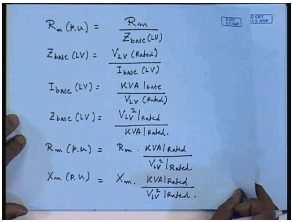Now, if we want to convert them into per unit then R m per unit will be R m divided by z base of the LV side, and z base of the LV side equal to V of the transformer LV side rated divided by I base of the LV side. Now I base of the LV side equal to KVA base divided by LV side voltage base. Therefore z base LV side equal to V LV rated square divided by KVA rated. Hence R e mu will be equal to R m into KVA rated KVA of the transformer divided by rated LV voltage square.

Similarly X m per unit would be X m into rated KVA of the transformer divided to a V LV square rated. So, thus we can find out the actual shunt branch parameter of the transformer referred to the LV side and convert them to per unit parameter with LV side base quantities. So, the parameters of the equivalent circuit of a transformer approximate equivalent circuit parameters we have found R 0 and X m. Next we have to find out the parameters R e q and X e q, and this is done by short circuit test.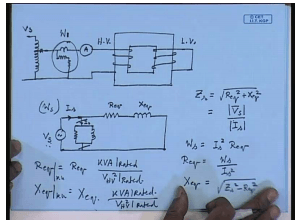In short circuit test what we do? We take the single phase transformer and short one of the sides. On the other side again we connect the ammeter, we connect a wattmeter and supply this again from a variac. It can be the same variac or some other variac. Again as before in the case of no load test question arises, which side should be shorted and which side the supply should be applied? Now the requirement for short circuit test is that it should be performed at rated current which means the ammeter should read the rated current of the side it is connected. Obviously, if this is the LV side then we will have to supply a very large current, because LV side rated current is much larger than the HV side rated current. Hence normally the short circuit test is performed on the HV side.

So, this is the HV side and the LV side is shorted. Again coming to the question of wattmeter, the wattmeter current coil now should be capable of carrying the rated HV side current; however, the voltage required to circulate rated HV side current when the LV side is short circuited is very small. It is usually between 5 to 10 percent of the rated HV side voltage, and that is another reason why the short circuit test is performed on the HV side, because one does not need to apply the full HV voltage; only very small fraction of the HV voltage is required. But the wattmeter potential coil should be sensitive enough to give accurate reading at that small voltage.

At no load we have said that the power factor is very poor; it is closed to 0. At short circuit power factor is still poor but may not be as bad as that in the case of an open circuit case. Hence a normal wattmeter can possibly be used, but in some very special cases this test may also require a LPF wattmeter, but we will assume a normal wattmeter will do. Now how do we find out the series branch parameter from the short circuit test? For that let us draw the approximate equivalent circuit under short circuit condition. Now this is the equivalent winding resistance, and this is the equivalent leakage resistance. Now refer to the HV side because the supply is applied to the HV side, and this is the magnetization branch again referred to the HV side.

At this point let us say this is the voltage applied V, short circuit I short circuit, and the wattmeter reading is w short circuit. Now even at when V s is at it is rated value we have seen the no load current I 0 is 5 to 7 percent of the rated current; that is I 0 if it is connected to the HV side then even if we apply rated voltage then I 0 is only 5 to 7 percent of I s. Therefore, when we reduce V s in order to and short the low voltage side we restrict I s to its rated value. The voltage that will be required for that we will send very negligible current through the magnetization branch, and hence for all practical purpose the magnetization branch can be neglected during short circuit; then we have very simple relation.

The short circuit impedance z s equal to square root of R e q square plus X e q square. This is given by V short circuit divided by I short circuit. The power loss during short circuit is assumed to be entirely due to the series resistance; therefore, W s equal to I s square R e q. Hence R e q equal to W s divided by I s square. Hence X e q equal to square root z s square minus R e q square. Just as in this R e q and X e q are now found referred to the HV side. Please remember the shunt branch parameters r m and j x m were found referred to the LV side. It is possible to either refer r m x m to the HV side or R e q and X e q to the LV side by the appropriate turns ratio and arrive at a complete approximate equivalent circuit of the transformer referred to either HV side or LV side.

However as I have mentioned earlier it is customary to represent this parameter in per unit and R e q, for example, can be represented in per unit as R e q that we have got from the test into KVA rated of the transformer divided by V HV square now rated HV voltage square. Similarly, X e q in per unit will be the value obtained from the test into KVA rated of the transformer divided by rated H V voltage square of the transformer.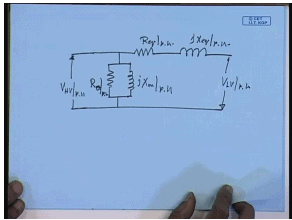Hence the approximate equivalent circuit of the transformer in per unit will look like.

Offer running on EduRev: Apply code STAYHOME200 to get INR 200 off on our premium plan EduRev Infinity!

61 docs|20 tests

,

,

,

,

,

,

,

,

,

,

,

,

,

,

,

,

,

,

,

,

,

;